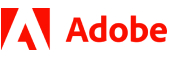New update is available. Click here to update.
Topics

# Anagram Difference

Moderate0/80
Average time to solve is 10m+1 more companies

## Problem statement

You have been given two strings, let's say 'STR1' and 'STR2' of equal lengths. You are supposed to return the minimum number of manipulations required to make the two strings anagrams.

Note:
``````An Anagram is a word or phrase formed by rearranging the letters of a different word or phrase. We can generalise this in string processing by saying that an anagram of a string is another string with the same quantity of each character in it, in any order.
``````
Example:
``````String “eat” and “ate” are anagram to each other but string “buy” and “bye” are not.
``````
Detailed explanation ( Input/output format, Notes, Images )
Constraints :
``````1<= T <= 100
1<= N <= 5*10^3

Where 'N' is the length of strings 'STR1' and 'STR2'.

Time limit: 1 sec
``````
Sample Input 1:
``````2
except
accept
bye
``````
Sample Output 1 :
``````2
1
``````
Explanation Of Sample Output 1 :
``````In test case 1, we can change two character of  'STR1' i.e. {'e','x'} to {'a','c'} or we can change two character of  'STR2' i.e. {'a','c'} to {'e','x'}, to make string anagram. So the minimum number of manipulations to make 'STR1' and  'STR2' to anagram string will be 2.

In test case 2, we can change one character of  'STR1' i.e. {'u'} to {'e'} or we can change one character of  'STR2' i.e. {'e'} to {'u'}, to make string anagram. So the minimum number of manipulations to make  'STR1' and 'STR2' to anagram string will be 1.
``````
Sample Input 2:
``````2
mail
male
ninja
ninja
``````
Sample Output 2 :
``````1
0
``````
Explanation Of Sample Output 2 :
``````In test case 1, we can change one character of  'STR1' i.e. {'i'} to {'e'} or we can change one character of  'STR2' i.e. {'e'} to {'i'}, to make string anagram. So the minimum number of manipulations to make  'STR1' and  'STR2' to anagram string will be 1.

In test case 2, both strings are already anagram. So we do not need to do any manipulation. So the minimum number of manipulations to make  'STR1' and  'STR2' to anagram string will be 0.
``````Console